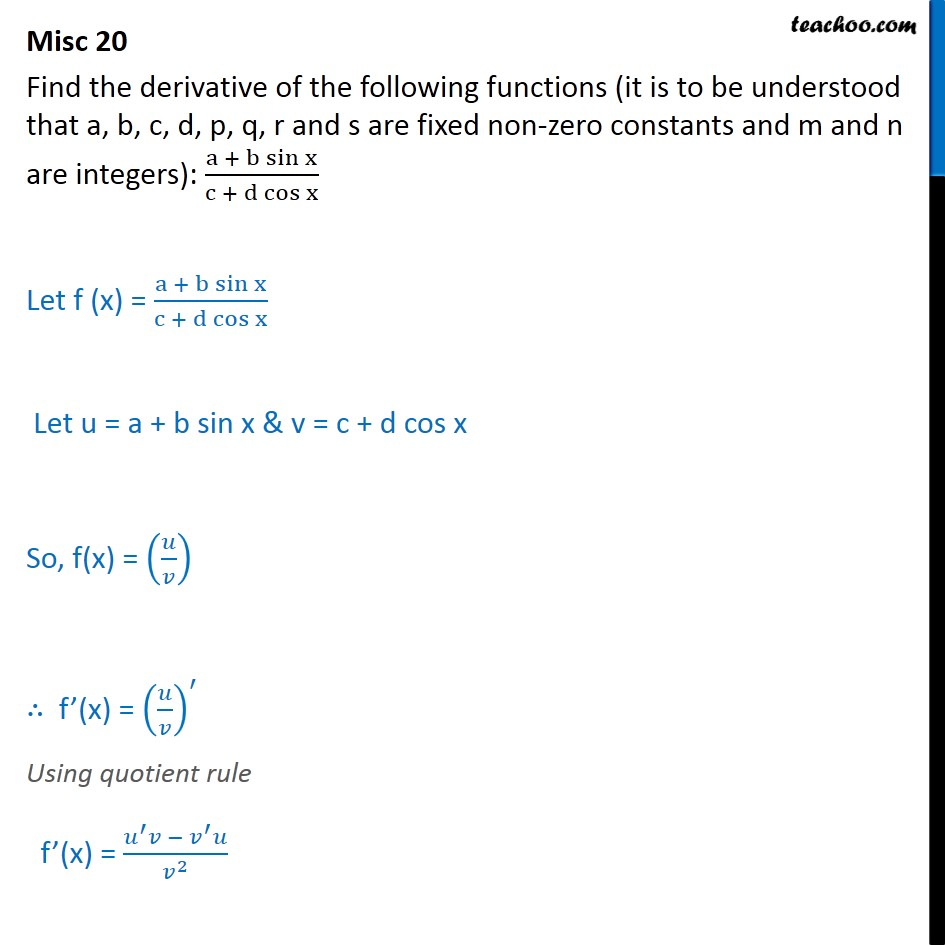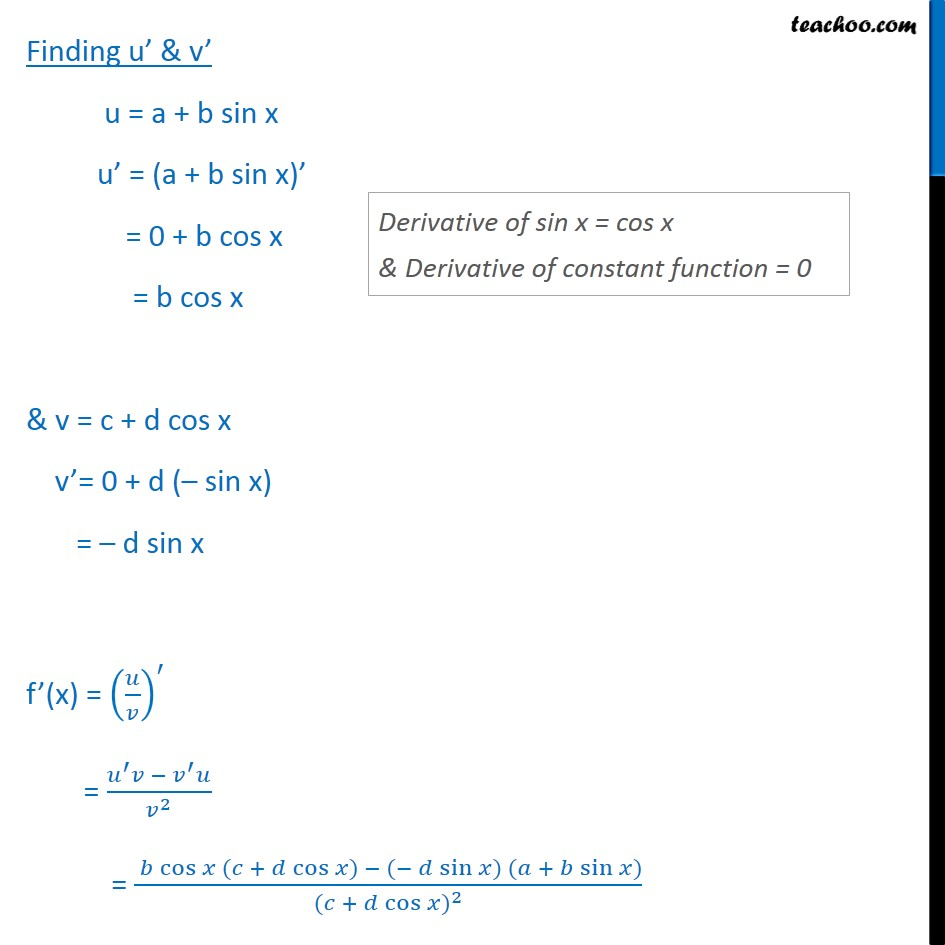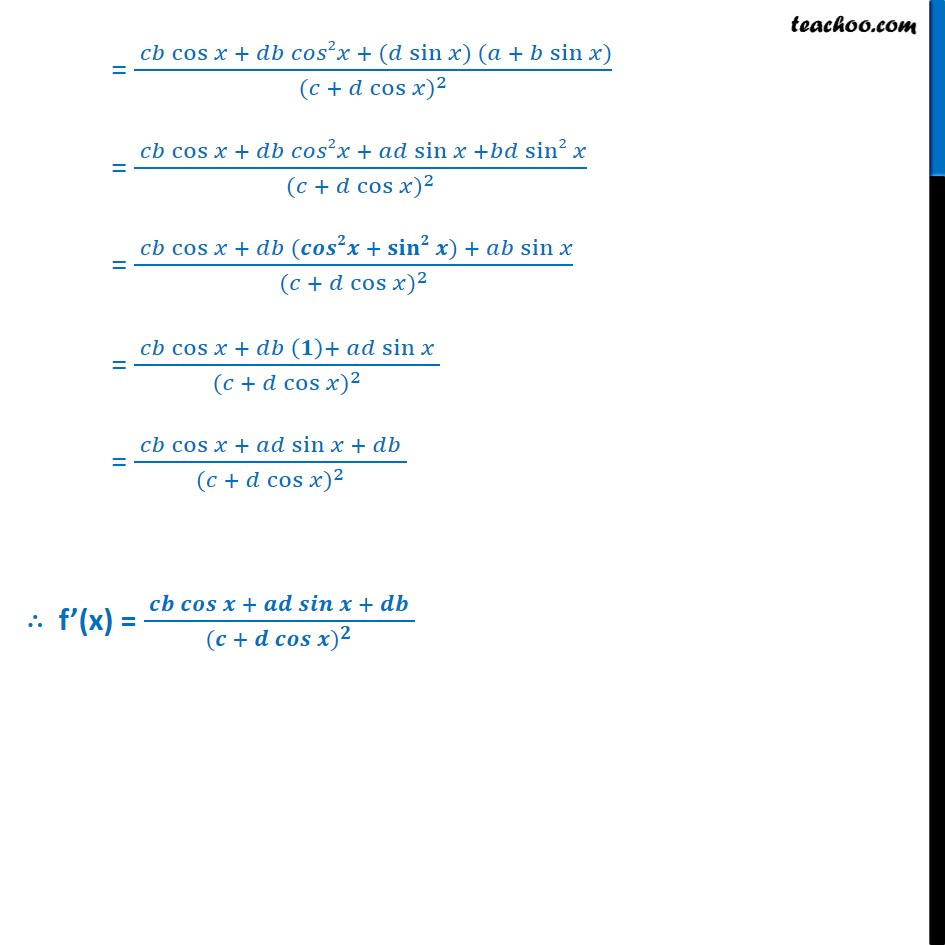Miscellaneous (Term 1 and Term 2)

Chapter 13 Class 11 Limits and Derivatives (Term 1 and Term 2)
Serial order wise### Transcript

Misc 20 Find the derivative of the following functions (it is to be understood that a, b, c, d, p, q, r and s are fixed non-zero constants and m and n are integers): a + b sin﷮x﷯﷮c + d cos x﷯ Let f (x) = a + b sin﷮x﷯﷮c + d cos x﷯ Let u = a + b sin x & v = c + d cos x So, f(x) = 𝑢﷮𝑣﷯﷯ ∴ f’(x) = 𝑢﷮𝑣﷯﷯﷮′﷯ Using quotient rule f’(x) = 𝑢﷮′﷯𝑣 − 𝑣﷮′﷯𝑢﷮ 𝑣﷮2﷯﷯ Finding u’ & v’ u = a + b sin x u’ = (a + b sin x)’ = 0 + b cos x = b cos x & v = c + d cos x v’= 0 + d (– sin x) = – d sin x f’(x) = 𝑢﷮𝑣﷯﷯﷮′﷯ = 𝑢﷮′﷯𝑣 − 𝑣﷮′﷯𝑢﷮ 𝑣﷮2﷯﷯ = 𝑏 cos﷮𝑥 (𝑐 + 𝑑 cos﷮𝑥) − (− 𝑑 sin﷮𝑥) (𝑎 + 𝑏 sin﷮𝑥)﷯﷯﷯﷯﷮ (𝑐 + 𝑑 cos﷮𝑥﷯)﷮2﷯﷯ = 𝑐𝑏 cos﷮𝑥 + 𝑑𝑏 𝑐𝑜𝑠2𝑥 + (𝑑 sin﷮𝑥) (𝑎 + 𝑏 sin﷮𝑥)﷯﷯﷯﷮ (𝑐 + 𝑑 cos﷮𝑥﷯)﷮2﷯﷯ = 𝑐𝑏 cos﷮𝑥 + 𝑑𝑏 𝑐𝑜𝑠2𝑥 + 𝑎𝑑 sin﷮𝑥 +𝑏𝑑 sin2﷮𝑥﷯﷯﷯﷮ (𝑐 + 𝑑 cos﷮𝑥﷯)﷮2﷯﷯ = 𝑐𝑏 cos﷮𝑥 + 𝑑𝑏 (𝒄𝒐𝒔𝟐𝒙 + 𝐬𝐢𝐧𝟐﷮𝒙﷯) + 𝑎𝑏 sin﷮𝑥﷯﷯﷮ (𝑐 + 𝑑 cos﷮𝑥﷯)﷮2﷯﷯ = 𝑐𝑏 cos﷮𝑥 + 𝑑𝑏 𝟏﷯+ 𝑎𝑑 sin﷮𝑥﷯ ﷯﷮ (𝑐 + 𝑑 cos﷮𝑥﷯)﷮2﷯﷯ = 𝑐𝑏 cos﷮𝑥 + 𝑎𝑑 sin﷮𝑥 ﷯+ 𝑑𝑏 ﷯﷮ (𝑐 + 𝑑 cos﷮𝑥﷯)﷮2﷯﷯ ∴ f’(x) = 𝒄𝒃 𝒄𝒐𝒔﷮𝒙 + 𝒂𝒅 𝒔𝒊𝒏﷮𝒙 ﷯+ 𝒅𝒃 ﷯﷮ (𝒄 + 𝒅 𝒄𝒐𝒔﷮𝒙﷯)﷮𝟐﷯﷯